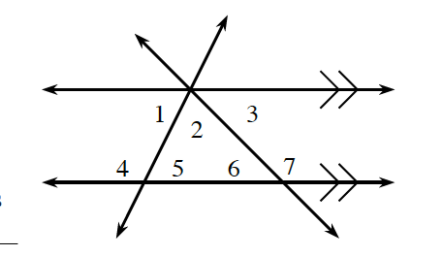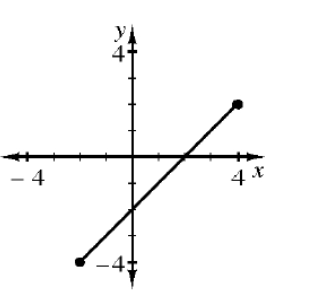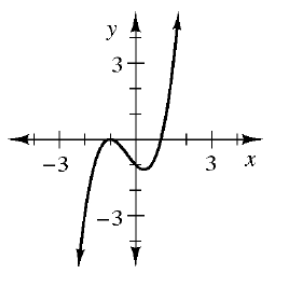9.1.1
starstarstarstarstarstarstarstarstarstar
by Kathleen Lucchesi
| 12 Questions
Note from the author:
CPM CC3 assignments by lesson1
1

A

B

C

D2
1

A

B

C

D3
5
If  measure of angle 1 = 74º and  measure of angle 4 = 3x – 18º, write an equation and find  x.4
2
If  measure of angle 2 = 3x – 9º and  measure of angle 1 = x + 25º, write an equation to find  x.  Then find  measure of angle 2.5
10
If measure of angle 5 = 53˚  and  measure of angle 7 = 125˚, find the measures of each numbered angle.
6
1
Josue called his father to say that he was almost home.  He had traveled 61.5 miles, which was 3/4 of the way home.  Write and solve an equation to calculate the total distance he will travel to get home.7
1
The image above is a function.
True
False8
1
The image above is a function.
True
False9
1
The image above is a function.
True
False10
1
The image above is a function.
True
False11
1
The image above is a function.
True
False12
1
The image above is a function.
True
False